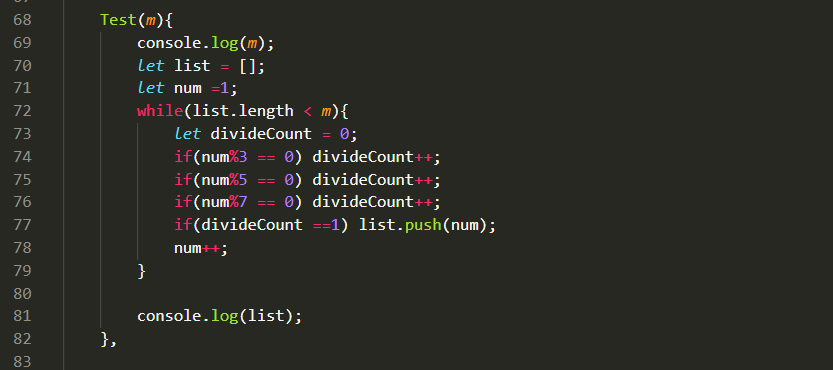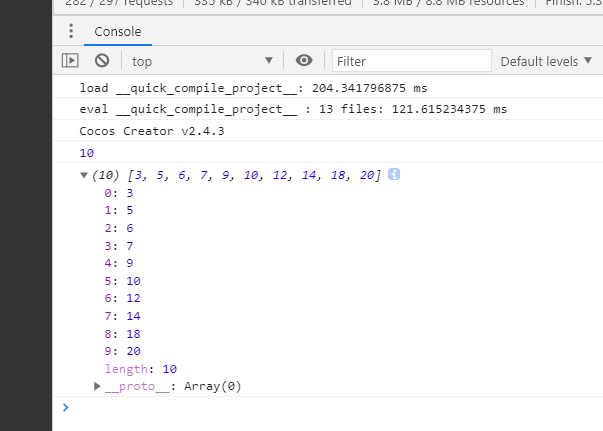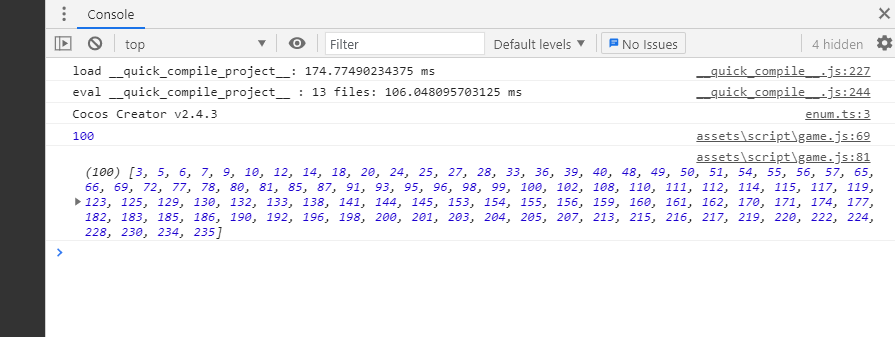2021-05-28 17:35

# 求c语言大佬帮帮孩子吧

c语言编写函数，求出m个可以被3、5或7整除，但不能被其中两个同时整除的所有整数从小到大放在数组中。在main函数中验证。要求：m在main函数中输入，数组在main函数中输出。

• 写回答
• 好问题 提建议
• 关注问题
• 收藏
• 邀请回答

#### 2条回答默认 最新

•转接R 2021-05-28 21:05
已采纳
``````#include<stdio.h>
#define MAXNUM 10//输出个数
bool judge(int i){
int a=0;
if(i%3==0)
a++;
if(i%5==0)
a++;
if(i%7==0)
a++;
if(a==1)
return 1;
else
return 0;
}
int main(){
int num[MAXNUM];
int i=1;
int n=0;
while(1){
if(n==MAXNUM){
break;
}
if(judge(i)){
num[n]=i;
n++;
}
i++;
}
for(int j=0;j<MAXNUM;j++){
printf("%d ",num[j]);
}
return 0;
}``````
已采纳该答案
评论
解决 无用
打赏 举报
•方法给你

评论
解决 无用
打赏 举报